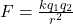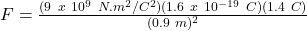Question

A proton is 0.9 meters away from a 1.4 C charge. What is the magnitude of the electric force between the proton and the charge

1.F = 2.49 x 10⁻⁹ N

Explanation:

The electrostatic force between two charged bodies is given by Colomb’s Law:where,

F = Electrostatic Force = ?

k = colomb’s constant = 9 x 10⁹ N.m²/C²

q₁ = charge on proton = 1.6 x 10⁻¹⁹ C

q₂ = second charge = 1.4 C

r = distace between charges = 0.9 m

Therefore,F = 2.49 x 10⁻⁹ N

2.